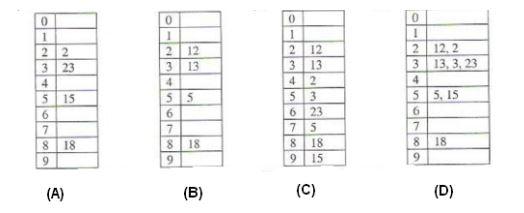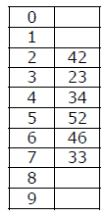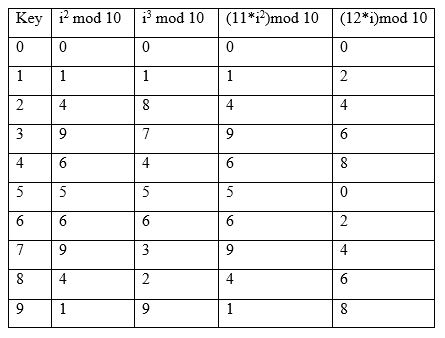# Practice Problems on Hashing

• Difficulty Level : Easy
• Last Updated : 08 Jul, 2021

In this article, we will discuss the types of questions based on hashing. Before understanding this, you should have idea about hashing, hash function, open addressing and chaining techniques (see: Introduction, Separate chaining, Open addressing).

These are some key points in hashing:

Attention reader! Don’t stop learning now.  Practice GATE exam well before the actual exam with the subject-wise and overall quizzes available in GATE Test Series Course.

Learn all GATE CS concepts with Free Live Classes on our youtube channel.

• The purpose of hashing is to achieve search, insert and delete an element in complexity O(1).
• Hash function is designed to distribute keys uniformly over the hash table.
• Load factor α in hash table can be defined as number of slots in hash table to number of keys to be inserted.
• For open addressing, load factor α is always less than one.
• The complexity of insertion, deletion and searching using open addressing is 1/(1-α).
• The complexity of insertion, deletion and searching using chaining method is (1+α).

These are the types of questions asked in hashing.

Type 1: Calculation of hash values for given keys –
In this type of questions, hash values are computed by applying given hash function on given keys.

Que – 1. Given the following input (4322, 1334, 1471, 9679, 1989, 6171, 6173, 4199) and the hash function x mod 10, which of the following statements are true? (GATE CS 2004)
i. 9679, 1989, 4199 hash to the same value
ii. 1471, 6171 hash to the same value
iii. All elements hash to the same value
iv. Each element hashes to a different value
(A) i only
(B) ii only
(C) i and ii only
(D) iii or iv

Solutions: Using given hash function h(x) = x mod 10

```h(9679) = 9679%10 = 9
h(1989) = 1989%10 = 9
h(4199) = 4199%10 = 9
h(1471) = 1471%10 = 1
h(6171) = 6171%10 = 1 ```

As we can see, 9679, 1989 and 4199 hash to same value 9. Also, 1471 and 6171 hash to same value 1. Therefore, statement (i) and (ii) are correct which match with option (C).

Type 2: Insertion of keys into hash table using linear probing as collision resolution technique –
In linear probing technique, collision is resolved by searching linearly in the hash table until an empty location is found.

Que – 2. The keys 12, 18, 13, 2, 3, 23, 5 and 15 are inserted into an initially empty hash table of length 10 using open addressing with hash function h(k) = k mod 10 and linear probing. What is the resultant hash table?Solution: Keys 12, 18, 13, 2, 3, 23, 5 and 15 are inserted in hash table as:

For key 12, h(12) is 12%10 = 2. Therefore, 12 is placed at 2nd index in the hash table.
For key 18, h(18) is 18%10 = 8. Therefore, 18 is placed at 8th index in the hash table.
For key 13, h(13) is 13%10 = 3. Therefore, 13 is placed at 3rd index in the hash table.
For key 2, h(2) is 2%10 = 2. However, index 2 is already occupied with 12. Therefore, using linear probing, 2 will be placed at index 4 as index 2 and 3 are already occupied.
For key 3, h(3) is 3%10 = 3. However, index 3 is already occupied with 13. Therefore, using linear probing, 3 will be placed at index 5 as index 3 and 4 are already occupied.
Similarly, 23, 5 and 15 will be placed at index 6, 7, 9 respectively.

Therefore, correct option is (C).

Alternative Approach: We can also solve this using elimination approach as:

Option (A) and (B) are incorrect as all keys are not inserted in hash table.
Option (D) is incorrect as some indexes in hash table have more than one key which never happens using linear probing.
Remaining option is (C) which is the answer.

Type 3: Given a hash table with keys, verify/find possible sequence of keys leading to hash table –
For a given hash table, we can verify which sequence of keys can lead to that hash table. However, to find possible sequences leading to a given hash table, we need to consider all possibilities.

Que – 3. A hash table of length 10 uses open addressing with hash function h(k)=k mod 10, and linear probing. After inserting 6 values into an empty hash table, the table is as shown below.Which one of the following choices gives a possible order in which the key values could have been inserted in the table?
(A) 46, 42, 34, 52, 23, 33
(B) 34, 42, 23, 52, 33, 46
(C) 46, 34, 42, 23, 52, 33
(D) 42, 46, 33, 23, 34, 52

Solution: We will check whether sequence given in option A can lead to hash table given in question. Option A inserts 46, 42, 34, 52, 23, 33 as:

For key 46, h(46) is 46%10 = 6. Therefore, 46 is placed at 6th index in the hash table.
For key 42, h(42) is 42%10 = 2. Therefore, 42 is placed at 2nd index in the hash table.
For key 34, h(34) is 34%10 = 4. Therefore, 34 is placed at 4th index in the hash table.
For key 52, h(52) is 52%10 = 2. However, index 2 is occupied with 42. Therefore, 52 is placed at 3rd index in the hash table. But in given hash table, 52 is placed at 5th index. Therefore, sequence in option A can’t generate hash table given in question.

In the similar way, we can check for other options as well which leads to answer as (C).

Que – 4. How many different insertion sequences of the key values using the same hash function and linear probing will result in the hash table given in Question 3 above?
(A) 10
(B) 20
(C) 30
(D) 40

Solution: The first key which is not at the index computed by hash function is 52. It means index 2, 3 and 4 were already occupied and therefore, key 52 is placed at index 5.

The keys 42, 23 and 34 are present at index 2, 3, and 4 respectively. As these keys are at their correct position, their order of insertion does not matter. These 3 keys can be inserted in 3! = 6 ways. Therefore, the sequence will be any order of (42, 23, 34) followed by 52.

The next key which is not at the index computed by hash function is 33. It means indexes 3 to 6 were already occupied and key 33 is placed at index 7. Therefore, it is the last key to be inserted into hash table.

The key 46 is present at its correct position computed by hash function. Therefore, it can be inserted at any place in the sequence before 33. The sequence excluding 33 has 4 elements 42, 23, 34, 52 which create 5 positions for 46 (3 in-between and 2 corners).
Total number of ways is: 6*5 =30

Type 4: Chaining based collision resolution technique –
In chaining based collision resolution technique, the keys generating same hash value are placed in same bucket using pointers. The different types of questions based on chaining technique are:

Que – 5. Consider a hash table with 100 slots. Collisions are resolved using chaining. Assuming simple uniform hashing, what is the probability that the first 3 slots are unfilled after the first 3 insertions? (GATE-CS-2014)
(A) (97 × 97 × 97)/100^3
(B) (99 × 98 × 97)/100^3
(C) (97 × 96 × 95)/100^3
(D) (97 × 96 × 95)/(3! × 100^3)

Solution: In uniform hashing, the function evenly distributes keys into slots of hash table. Also, each key has an equal probability of being placed into a slot, being independent of the other elements already placed.

Therefore, the probability of remaining first 3 slots empty for first insertion (choosing 4 to 100 slot) = 97/100. As next insertions are independent on previous insertion, the probability for next insertions will also be 97/100. The required probability will be (97/100)^3.

Que – 6. Which one of the following hash functions on integers will distribute keys most uniformly over 10 buckets numbered 0 to 9 for i ranging from 0 to 2020? (GATE-CS-2015)
(A) h(i) =i^2 mod 10
(B) h(i) =i^3 mod 10
(C) h(i) = (11 ∗ i^2) mod 10
(D) h(i) = (12 ∗ i) mod 10

Solution: In uniform distribution, the function evenly distributes keys into slots of hash table.
For given hash functions, we have calculated hash values for keys 0 to 9 as:As we can see from the table, i^3 mod10 is distributing evenly from indexes 0 to 9. Other functions have not utilized all indexes.

My Personal Notes arrow_drop_up